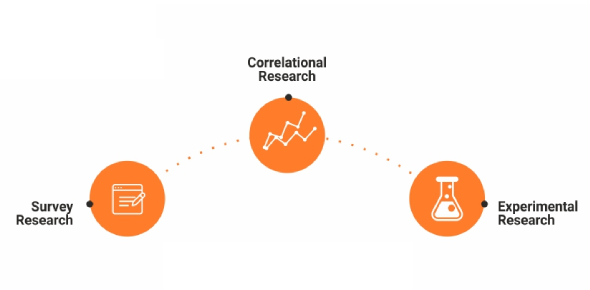# Quiz: Quantitative Research In ELT

12 Questions | Total Attempts: 26Settings.

• 1.
An abstract is “a concise summary of the entire paper.”
• A.

True

• B.

False

• 2.
Informative abstract describes the major points of the project to the reader. Includes the background, purpose and focus of the paper or article, but never the methods, results and conclusions, if it is a research paper.
• A.

True

• B.

False

• 3.
A gap statement is found in the Introduction section of a journal article or poster or in the Goals and Importance section of a research proposal and succinctly identifies for your audience the gap that you will attempt to address in your project.
• A.

True

• B.

False

• 4.
literature review is a survey of scholarly sources (such as books, journal articles, and theses) related to a specific topic or research question. It is often written as part of a thesis, dissertation, or research paper, in order to situate your work in relation to existing knowledge
• A.

True

• B.

False

• 5.
Pre-experiments are the simplest form of research design. In a pre-experiment either a single group or multiple groups are observed subsequent to some agent or treatment presumed to cause change.
• A.

True

• B.

False

• 6.
quasi-experiment is an empirical interventional study used to estimate the causal impact of an intervention on target population without random assignment.
• A.

True

• B.

False

• 7.
Survey Research is defined as the process of conducting research using surveys that researchers send to survey respondents. The data collected from surveys is then statistically analyzed to draw meaningful research conclusions
• A.

True

• B.

False

• 8.
Correlational research is a type of non-experimental research method in which a researcher measures two variables, understands and assesses the statistical relationship between them with no influence from any extraneous variable.
• A.

True

• B.

False

• 9.
In statistics, normality tests are used to determine if a data set is well-modeled by a normal distribution and to compute how likely it is for a random variable underlying the data set to be normally distributed.
• A.

True

• B.

False

• 10.
Nominal data is a group of non-parametric variables, while Ordinal data is a group of non-parametric ordered variables
• A.

True

• B.

False

• 11.
The coefficient of reliability falls between 0 and 1, with perfect reliability equaling 1, and no reliability equaling 0. The general rule is that reliability greater than 0.8 are considered as high
• A.

True

• B.

False

• 12.
The purpose of the discussion is to interpret and describe the significance of your findings in light of what was already known about the research problem being investigated, and to explain any new understanding or fresh insights about the problem after you've taken the findings into consideration
• A.

True

• B.

False

Related TopicsBack to top